International
Tables for
Crystallography
Volume A
Space-group symmetry
Edited by M. I. Aroyo

International Tables for Crystallography (2016). Vol. A, ch. 3.1, pp. 698-700

Section 3.1.1. Bases and lattices

H. Burzlaffa and H. Zimmermannb

3.1.1. Bases and lattices

| top | pdf |

3.1.1.1. Description and transformation of bases

| top | pdf |

In three dimensions, a coordinate system is defined by an origin and a basis consisting of three non-coplanar vectors. The lengths a, b, c of the basis vectors a, b, c and the intervector angles,,are called the metric parameters. In n dimensions, the lengths are designatedand the angles, where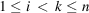.

Another description of the basis consists of the scalar products of all pairs of basis vectors. The set of these scalar products obeys the rules of covariant tensors of the second rank (see Section 1.5.2). The scalar products may be written in the form of amatrix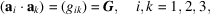which is called the matrix of the metric coefficients or the metric tensor.

The change from one basis to another is described by a transformation matrix P. The transformation of the old basis (abc) to the new basisis given by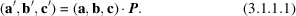The relation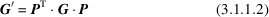holds for the metric tensors G and.

3.1.1.2. Lattices

| top | pdf |

A three-dimensional lattice can be visualized best as an infinite periodic array of points, which are the termini of the vectorsThe parallelepiped determined by the basis vectors a, b, c is called a (primitive) unit cell of the lattice (cf. Section 1.3.2.3), a, b and c are a primitive basis of the lattice. The number of possible lattice bases is infinite.

For the investigation of the properties of lattices, appropriate bases are required. In order to select suitable bases (see below), transformations may be necessary (Chapter 1.5). Of the several properties of lattices, only symmetry and some topological aspects are considered in this section. Some further properties of lattices are given in Section 3.1.4.

3.1.1.3. Topological properties of lattices

| top | pdf |

The treatment of the topological properties is restricted here to the consideration of the neighbourhood of a lattice point. For this purpose, the domain of influence (Wirkungsbereich, Dirichlet domain, Voronoi domain, Wigner–Seitz cell) (Delaunay, 1933) is introduced. The domain of a particular lattice point consists of all points in space that are closer to this lattice point than to any other lattice point or at most equidistant to it. To construct the domain, the selected lattice point is connected to all other lattice points. The set of planes perpendicular to these connecting lines and passing through their midpoints contains the boundary planes of the domain of influence, which is thus a convex polyhedron. (Niggli and Delaunay used the term domain of influence' for the interior of the convex polyhedron only.) Without the use of metrical properties, Minkowski (1897) proved that the maximal number of boundary planes resulting from this construction is equal to, where n is the dimension of the space. The minimal number of boundary planes is 2n. Each face of the polyhedron represents a lattice vector. Thus, the topological, metrical and symmetry properties of infinite lattices can be discussed with the aid of a single finite polyhedron, namely the domain of influence (cf. Burzlaff & Zimmermann, 1977).

3.1.1.4. Special bases for lattices

| top | pdf |

Different procedures are in use to select special bases of lattices. The reduction procedures employ metrical properties to develop a sequence of basis transformations which lead to a reduced basis and reduced cell (see Section 3.1.4).

Another possibility is to make use of the symmetry properties of lattices. This procedure, with the additional aid of standardization rules, leads to the conventional crystallographic basis and cell. In addition to translational symmetry, a lattice possesses point-group symmetry. No crystal can have higher point-group symmetry than the point group of its lattice, which is called its holohedry. (Detailed treatment of the symmetry properties of lattices and their classification is given in Chapter 1.3. Following the terminology introduced there, the lattice point groups are the geometric classes to which the Bravais groups of the lattices belong.) The seven holohedries in three dimensions and the four in two dimensions form the basis for the classification of lattices (Table 3.1.1.1). It may be shown by an algebraic approach (Burckhardt, 1966) or a topological one (Delaunay, 1933) that the arrangement of the symmetry elements with respect to the lattice vectors is not arbitrary but well determined. Taking as basis vectors lattice vectors along important symmetry directions and choosing the origin in a lattice point simplifies the description of the lattice symmetry operations (cf. Section 3.3.1). Note that such a basis is not necessarily a (primitive) basis of the lattice (see below). The choice of a basis controlled by symmetry is not always unique; in the monoclinic system, for example, one vector can be taken parallel to the symmetry direction but the other two vectors, perpendicular to it, are not uniquely determined by symmetry.

 Table 3.1.1.1| top | pdf | Lattice point-group symmetries
 (a) Two dimensions.
Lattice point group22mm4mm6mm
Crystal family m o t h
monoclinic (oblique) orthorhombic (rectangular) tetragonal (square) hexagonal
 (b) Three dimensions.
Lattice point groupCrystal family a m o t h c
anorthic (triclinic) monoclinic orthorhombic tetragonal hexagonal cubic
The symbols for crystal families were adopted by the International Union of Crystallography in 1985; cf. de Wolff et al. (1985).

The choice of conventions for standardizing the setting of a lattice depends on the purpose for which it is used. The several sets of conventions rest on two conflicting principles: symmetry considerations and metric considerations. The following rules (i) to (vii) defining a conventional basis are taken from Donnay (1943; Donnay & Ondik, 1973); they deal with the conventions based on symmetry:

 (i) Each basis vector is a lattice vector from the origin to the nearest node on the related row. The basis must define a right-handed coordinate system. (ii) The basis vectors for a cubic lattice are parallel to the fourfold axes. (iiia) In a hexagonal lattice, the basis vector parallel to the sixfold axis is labelled c. The remaining two basis vectors are taken along equivalent twofold axes and they must include an angle of; from the two possible sets, the shorter vectors are chosen. (iiib) For rhombohedral lattices, two descriptions are given in the present edition, as in earlier ones. The first description which results in the conventional cell uses hexagonal axes'. In this case, c is taken along the threefold axis. The remaining two vectors are chosen along equivalent twofold axes, which include an angle of; they are oriented so that lattice points occur atand(obverse setting). The reverse setting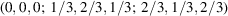is not used in the space-group tables (cf. the second footnote to Table 2.1.1.2). The second description uses rhombohedral axes': a, b and c are the shortest three non-coplanar lattice vectors symmetry-equivalent with respect to the threefold axis. (iv) In a tetragonal lattice, the vector c is along the fourfold axis, and a and b are chosen along equivalent twofold axes perpendicular to each other. From the two possible sets, the shorter vectors are chosen. (v) In an orthorhombic lattice, a, b and c must be taken along the three twofold axes. (vi) For monoclinic lattices, two settings' are given in the present edition. In one setting, the only symmetry direction is labelled b (b-unique setting). The basis vectors a and c are chosen to be the shortest two vectors in the net plane perpendicular to b, the angle β should be non-acute. This occurs if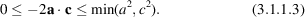In the other setting, the symmetry direction is labelled c [c-unique setting; first introduced in International Tables for X-ray Crystallography (1952)]. In this case, a and b are the shortest two vectors in the net plane perpendicular to c and the angle γ should be non-acute. The b-unique setting is considered to be the standard setting. (vii) The reduced basis is used to describe a triclinic (= anorthic) lattice (cf. Section 3.1.4).

The metric parameters of the conventional basis are called lattice parameters. For the purpose of identification, additional metric rules are to be applied to make the labelling unique; they can be found in the introduction to Crystal Data (Donnay & Ondik, 1973).

When the above rules have been applied, it may occur that not all lattice points can be described by integral coordinates. In such cases, the unit cell contains two, three or four lattice points. The additional points may be regarded as centrings of the conventional cell. They have simple rational coordinates. For a conventional basis, the number of lattice points per cell is 1, 2, 3 or 4 (see Tables 3.1.2.1and 3.1.2.2).

In two dimensions, only two centring types are needed: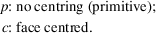In three dimensions, the following centring types are used: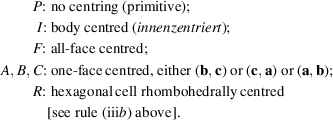In orthorhombic and monoclinic lattices, some differently centred cells can be transformed into each other without violating the symmetry conditions for the choice of the basis vectors. In these cases, the different centred cells belong to the same centring mode. In the orthorhombic case, the three types of one-face-centred cells belong to the same centring mode because the symbol of the cell depends only on the labelling of the basis vectors; C is usually preferred to A and B as the standard setting; the centring mode is designated S (seitenflächenzentriert). In the monoclinic case (b-unique setting), A, I and C may occur if the basis is chosen according to rule (vi). They can be transformed into each other without changing the symmetry direction. C is used for the standard setting (cf. Section 2.1.1.2); it represents the centring mode S. The vectors a, c are conventionally chosen as short as the C-centring allows so that they need not be the shortest two vectors in their net plane and need not fulfil the inequalities (3.1.1.3).

3.1.1.5. Remarks

| top | pdf |

 (i) For the handling of special problems such as subgroup relations, it may be convenient to use additional types of centred cells, e.g. a hexagonal cell centred atandwhich is called H. In this case, rule (iiia) above is violated as vectors a and b are now directed along the second set of equivalent twofold axes. Similarly, for tetragonal lattices, C may be used instead of P, or F instead of I; cf. Sections 1.5.4and 2.1.1.2. (ii) Readers who have studied Chapter 1.3may realize that the lattice bases' defined here are also called primitive bases' and that both primitive bases' and conventional bases' are special cases of bases used in crystallography.

References

International Tables for X-ray Crystallography (1952). Vol. I, edited by N. F. M. Henry & K. Lonsdale, pp. 530–535. Birmingham: Kynoch Press.
Buerger, M. J. (1957). Reduced cells. Z. Kristallogr. 109, 42–60.
Burckhardt, J. (1966). Die Bewegungsgruppen der Kristallographie, 2nd ed., pp. 82–89. Basel: Birkhäuser.
Burzlaff, H. & Zimmermann, H. (1977). Symmetrielehre. Part I of the series Kristallographie, pp. 96–135. Stuttgart: Thieme.
Delaunay, B. N. (1933). Neuere Darstellung der geometrischen Kristallographie. Z. Kristallogr. 84, 109–149.
Donnay, J. D. H. (1943). Rules for the conventional orientation of crystals. Am. Mineral. 28, 313–328, 470.
Donnay, J. D. H. & Ondik, H. M. (1973). Editors. Crystal Data, Vol. 2, 3rd ed. Introduction, p. 2. Washington: National Bureau of Standards.
Minkowski, H. (1897). Allgemeine Lehrsätze über die konvexen Polyeder. In Gesammelte Abhandlungen. Leipzig, 1911. [Reprinted: Chelsea, New York (1967).]